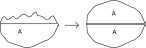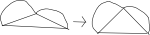# Numb3rs 214: Harvest

In this episode Charlie talks about surface tension in water and why it makes drops of water try to be spherical and puddles try to be circles. He also talks about optimization theory and how it applies to matching organ donors with people who need organs.

## The Isoperimetric Inequality

In the episode Charlie and Larry want to figure out when a crime was committed by determining how quickly a water puddle formed from melting ice would grow, and then using this along with the size of the puddle to ascertain when the ice started melting. To actually do this they would have to use some complicated differential equations which involve surface tension, rate of evaporation, temperature, humidity, the type of surface, etc. However, the most basic thing that keeps the water in a puddle is surface tension.
Experimenting with Surface Tension
Here are a few experiments you can do to see surface tension in action.
1. Get a penny and an eyedropper full of water. Slowly drop water onto the penny, one drop at a time (if you don't have an eyedropper, find some other way of doing this). Now make your eyes the same height as the penny and look at the water on it. After you add enough drops the water will spill off the penny. How many do you think it will take? Try it and find out. What is the shape of the water right before you add the last drop? Is it what you expected?
2. Get a test tube or some other skinny transparent tube that will hold water. Put some water in it and look closely at the surface. Is it flat? Why not?
3. For some other experiments and some explanations about surface tension, try looking here.

Now we can use surface tension to explain why puddles try to form circles. (Sometimes circles might not actually be formed because of irregularities in the surface or the way the drop was dropped.) Now imagine a drop of water on a surface. The surface tension will pull the drop together, and this will make the drop try to take on a shape which minimizes the surface area of the drop while maintaining the same volume. If we translate this condition into mathematics, we get the following questions. Given a particular area A in a plane, is there a shape with area A that has a minimal perimeter among all shapes with area A? What is that shape?

Activity 2*
Suppose that the answer to the first question is yes, and that the shape is C. Figure out why the following two statements are equivalent.
1. Among all planar shapes with the same perimeter the shape C has the largest area.
2. Among all planar shapes with the same area the shape C has the shortest perimeter.

The answer to the first question is yes, but to prove it some fairly advanced calculus-type arguments are needed. However, if we assume that such a shape exists, then there is a simple argument that shows the answer to the second question is a circle.

The argument has three steps to it. The first step is to show that the shape S must be convex. This means that given any two points on the boundary, or edge, of the shape, the line segment between these two points lies entirely in the shape. Suppose that S is not convex. Then there are two points P,Q, with the property that the line segment between them doesn't lie entirely inside of the shape S. Then we can move P,Q along the line segment until the line segment L between them lies entirely outside the shape S. Then we can reflect the segment of the boundary of S that lies between P and Q across L to get a new shape with the same perimeter as S but with larger area. This contradicts the assumption that S had the largest area among shapes with its perimeter. This means that S must be convex.The second step is to show the following statement. If P,Q are points on the boundary of S that divide the perimeter into two equal parts, then the line segment L between them divides the area into two equal parts. To show this, we can use another picture. If the area of S below L is bigger than the area above L, we can remove the half above L and replace it with a reflected image of the part below L, and this will increase the area of S while preserving its perimeter. (We know that this move is allowed and that it increases the area because we know that S is convex.)Activity 3*:Remember the SAS theorem from geometry: if two triangles ABC, DEF have length AB = length DE, length BC = length EF, and angle ABC = angle DEF, then they are congruent. Show that if you are given two side lengths AB and BC, then the angle ABC which maximizes the area of the triangle is 90 degrees.

For the final step, it is enough to show that if we look at all arcs with a fixed length and endpoints on a fixed line, the semicircle gives the largest area. To do this, let P,Q be the points where the arc A intersects the line. It is enough so show that if we pick any point R on the boundary of A, the angle PRQ is a right angle. Suppose we pick a point R where this is not true. Then if you have done the activity above, you know that if we slide P along the line until the angle at R (the top point of the triangle in the drawing) is a right angle, we have increased the area of the triangle. Also, we haven't changed the areas of either of the regions with a curved border.The corresponding statement in higher dimensions, that a sphere is the shape with the lowest surface area to volume ratio, is also true, although different methods are required to prove it. This is the reason why balloons are spheres when they are blown up and why rain drops are spheres (or they would be if they were in a vacuum). Some balloons aren't spheres when they're blown up, but that's because some parts of the balloon are thicker than others and resist stretching.

## The Stable Marriage Problem

Finding optimal matches between organ donors and organ recipients is a difficult problem that involves a lot of biology and math. However, all the the biology is centered on evaluating how good the matches are. If we assume that if we have one recipient and two donors then we can decide which donor organ is a better match, then we can translate the problem entirely into math. Another simplification we'll make at first is that we have the same number of donors and recipients all at the same time and that there won't be any future donors or recipients.

After we make these assumptions, the problem is mathematically the same as the Stable Marriage Problem. In this problem, we have n men and women and each man has a ranking of the women that he likes, i.e. man 1 likes woman 3 most, then woman 5, then woman 2, etc., and each woman has a similar list of the men. In this case we can (arbitrarily) call the donors the men and the recipients the women. The goal of the problem is to find a stable matching, which means a way of "marrying" the men and women in stable way. This means that there is no pair of a man and a woman who would both prefer to be married to each other instead of to their current spouse. It was proved in 1962 by David Gale and Lloyd Shapley that it is always possible to do this. The algorithm is pretty simple, but before we describe it you can try an exmaple.

The Stable Marriage Problem is related to several other problems in the field of combinatorics, which in general deals with finite objects, graphs, finite optimization problems, "counting", and other related topics.
Find Stable Marriages
Below are two tables giving each man's preferences and each woman's preferences. The first number in the list is the favorite of that person, so man 1 likes woman 1 the most and woman 3 the least.
 Man 1: 1 2 3 Man 2: 3 1 2 Man 3: 1 3 2

 Woman 1: 3 1 2 Woman 2: 2 3 1 Woman 3: 2 3 1

The algorithm consists of several rounds of "proposals". At the beginning, each man proposes to the first woman on his list. After this, there is what computer scientists call a "loop", which means that the next two steps are repeated until everyone is married.

• First, if any woman has received a proposal from a man that she prefers over the man she is currently married to (if any), she divorces the old man and remarries to the new better man.
• Second, if any man isn't married, he proposes to the first person on his list that he hasn't proposed to.

Activity 4*:
1. Use this algorithm to determine marriages for the above tables.
2. Show that this algorithm results in stable marriages.

You might have the impression that this algorithm seems sexist, since the males are doing all the proposing. If you have that impression, you are very correct. It isn't too hard to show that the results of this algorithm are optimal for the males and worst for the females in the following sense. If there is a stable marriage between a man M and a woman W, we'll call M and W achievable partners. Then the results are optimal for the males in the sense that every male is married to his favorite acheivable partner, and similarly every woman is married to her least favorite achievable partner. Now since we are applying this algorithm to organ donors and recipients, it would probably be a good idea to make the matchings optimal for the recipients.

We have made one large simplification that makes the problem a lot easier to solve. We were assuming that there is just one round of matching organs to recipients. However, in real life there is a constant stream of organs that are being donated and a constant stream of new recipients. This complicates things because there might be a recipient who has an ok match with an organ, but might get a better match in a few weeks. This makes it hard to decide whether or not to take the immediately available one or to wait for a better option. Also, some recipients need organs more urgently than others, and organs only stay fresh for a day or so (to the best of my knowledge). All these factors make assigning organs a very difficult job.